# Dodecahedron

﻿
Dodecahedron
Regular Dodecahedron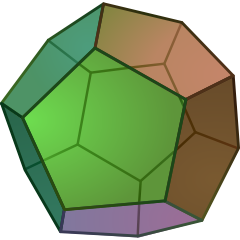Type Platonic solid
Elements F = 12, E = 30
V = 20 (χ = 2)
Faces by sides 12{5}
Schläfli symbol {5,3}
Wythoff symbol 3 | 2 5
Coxeter-DynkinSymmetry Ih, [5,3], (*532)
References U23, C26, W5
Properties Regular convex
Dihedral angle 116.56505° = arccos(-1/√5)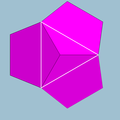5.5.5
(Vertex figure)Icosahedron
(dual polyhedron)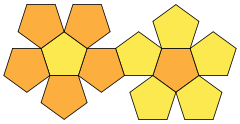Net

In geometry, a dodecahedron (Greek δωδεκάεδρον, from δώδεκα 'twelve' + εδρον 'base', 'seat' or 'face') is any polyhedron with twelve flat faces, but usually a regular dodecahedron is meant: a Platonic solid. It is composed of 12 regular pentagonal faces, with three meeting at each vertex, and is represented by the Schläfli symbol {5,3}. It has 20 vertices and 30 edges. Its dual polyhedron is the icosahedron, with Schläfli symbol {3,5}.

A large number of other (nonregular) polyhedra also have 12 sides, but are given other names. The most frequently named other dodecahedron is the rhombic dodecahedron.

## Dimensions

If the edge length of a regular dodecahedron is a, the radius of a circumscribed sphere (one that touches the dodecahedron at all vertices) is$r_u = \frac{a}{4} \left(\sqrt{15} +\sqrt{3}\right) \approx 1.401258538 \cdot a$

and the radius of an inscribed sphere (tangent to each of the dodecahedron's faces) is$r_i = \frac{a}{20} \sqrt{250 +110\sqrt{5}} \approx 1.113516364 \cdot a$

while the midradius, which touches the middle of each edge, is$r_m = \frac{a}{4} \left(3 +\sqrt{5}\right) \approx 1.309016994 \cdot a$

These quantities may also be expressed as$r_u = \frac{\sqrt{3}}{2} \phi \, a$$r_i = \frac{\phi^2}{2 \sqrt{3-\phi}} \, a \,\!$$r_m = \frac{\phi^2}{2} a$

where$\phi \,\!$ is the golden ratio.

Note that, given a regular pentagonal dodecahedron of edge length one,$r_u \,\!$ is the radius of a circumscribing sphere about a cube of edge length$\phi \,\!$, and$r_i \,\!$ is the apothem of a regular pentagon of edge length$\phi \,\!$.

## Area and volume

The surface area A and the volume V of a regular dodecahedron of edge length a are:$A = 3\sqrt{25+10\sqrt{5}} a^2 \approx 20.645728807a^2$$V = \frac{1}{4} (15+7\sqrt{5}) a^3 \approx 7.6631189606a^3$

## Cartesian coordinates

The following Cartesian coordinates define the vertices of a dodecahedron centered at the origin:

(±1, ±1, ±1)
(0, ±1/φ, ±φ)
(±1/φ, ±φ, 0)
(±φ, 0, ±1/φ)

where φ = (1+√5)/2 is the golden ratio (also written τ) = ~1.618. The edge length is 2/φ = √5–1. The containing sphere has a radius of √3.

## Properties

• The dihedral angle of a dodecahedron is 2 arctan(φ) or approximately 116.565 degrees.
• If the original dodecahedron has edge length 1, its dual icosahedron has edge length$\frac{1+\sqrt{5}}{2}$, the golden ratio.
• If we build the five Platonic solids with same volume, the dodecahedron has the shortest edges.
• It has 43,380 nets.
• The map-coloring number of a regular dodecahedron's faces is 4.
• The distance between the vertices on the same face not connected by an edge is φ.

## Geometric relations

The regular dodecahedron is the third in an infinite set of truncated trapezohedra which can be constructed by truncating the two axial vertices of a pentagonal trapezohedron.

The stellations of the dodecahedron make up three of the four Kepler-Poinsot polyhedra.

A rectified dodecahedron forms an icosidodecahedron.

The regular dodecahedron has 120 symmetries, forming the group$A_5\times Z_2$.

### Icosahedron vs dodecahedron

When a dodecahedron is inscribed in a sphere, it occupies more of the sphere's volume (66.49%) than an icosahedron inscribed in the same sphere (60.54%).

A regular dodecahedron with edge length 1 has more than three and a half times the volume of an icosahedron with the same length edges (7.663... compared with 2.181...).

A Regular Dodecahedron has fewer faces than the icosahedron, but more vertices.

## Related polyhedra

The dodecahedron can be transformed by a truncation sequence into its dual, the icosahedron:

 Picture Coxeter-DynkinDodecahedron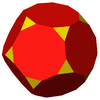Truncated dodecahedron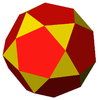Icosidodecahedron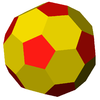Truncated icosahedronIcosahedronThe regular dodecahedron is a member of a sequence of otherwise non-uniform polyhedra and tilings, composed of pentagons with face configurations (V3.3.3.3.n). (For n>6, the sequence consists of tilings of the hyperbolic plane.) These face-transitive figures have (n32) rotational symmetry.

### Vertex arrangement

The dodecahedron shares its vertex arrangement with four nonconvex uniform polyhedra and three uniform polyhedron compounds.

Five cubes fit within, with their edges as diagonals of the dodecahedron's faces, and together these make up the regular polyhedral compound of five cubes. Since two tetrahedra can fit on alternate cube vertices, five and ten tetrahedra can also fit in a dodecahedron.

### Stellations

The 3 stellations of the dodecahedron are all regular (nonconvex) polyhedra: (Kepler-Poinsot polyhedra)

## Other dodecahedra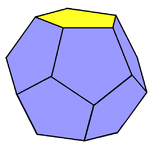The truncated pentagonal trapezohedron has d5d dihedral symmetry.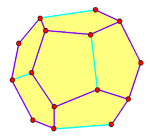The pyritohedron has th tetrahedral symmetry.

The term dodecahedron is also used for other polyhedra with twelve faces, most notably the rhombic dodecahedron which is dual to the cuboctahedron (an Archimedean solid) and occurs in nature as a crystal form. The Platonic solid dodecahedron can be called a pentagonal dodecahedron or a regular dodecahedron to distinguish it. The pyritohedron is an irregular pentagonal dodecahedron.

Other topologically distinct dodecahedra include:

In all there are 6,384,634 topologically distinct dodecahedra.

## History and uses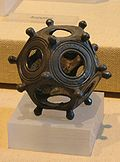Roman dodecahedronOmnidirectional Sound Source

Dodecahedral objects have found some practical applications, and have also played a role in the visual arts and in philosophy.

Plato's dialogue Timaeus (c. 360 B.C.) associates the other four platonic solids with the four classical elements, adding that "there is a fifth figure (which is made out of twelve pentagons), the dodecahedron—this God used as a model for the twelvefold division of the Zodiac." Aristotle postulated that the heavens were made of a fifth element, aithêr (aether in Latin, ether in American English), but he had no interest in matching it with Plato's fifth solid.

A few centuries later, small, hollow bronze Roman dodecahedra were made and have been found in various Roman ruins in Europe. Their purpose is not certain.

In twentieth century art, dodecahedra appear in the work of M. C. Escher, such as his lithograph Reptiles (1943), and in his Gravitation. In Salvador Dalí's painting The Sacrament of the Last Supper (1955), the room is a hollow dodecahedron.

In modern role-playing games, the dodecahedron is often used as a twelve-sided die, one of the more common polyhedral dice. Some quasicrystals have dodecahedral shape (see figure). Some regular crystals such as garnet and diamond are also said to exhibit "dodecahedral" habit, but this statement actually refers to the rhombic dodecahedron shape.

The popular puzzle game Megaminx is in the shape of a dodecahedron.

In the children's novel The Phantom Tollbooth, the Dodecahedron appears as a character in the land of Mathematics. Each of his faces wears a different expression – e.g. happy, angry, sad – which he swivels to the front as required to match his mood.

### Shape of the Universe

Various models have been proposed for the global geometry of the universe. In addition to the primitive geometries, these proposals include the Poincaré dodecahedral space, a positively curved space consisting of a dodecahedron whose opposite faces correspond (with a small twist). This was proposed by Jean-Pierre Luminet and colleagues in 2003 and an optimal orientation on the sky for the model was estimated in 2008.

## As a graph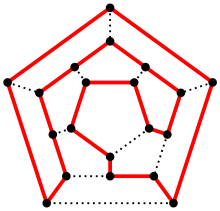A Hamiltonian cycle in a dodecahedron.

The skeleton of the dodecahedron – the vertices and edges – form a graph. The high degree of symmetry of the polygon is replicated in the properties of this graph, which is distance-transitive, distance-regular, and symmetric. The automorphism group has order 120. The vertices can be colored with 3 colors, as can the edges, and the diameter is 5.

The dodecahedral graph is Hamiltonian – there is a cycle containing all the vertices. Indeed, this name derives from a mathematical game invented in 1857 by William Rowan Hamilton, the icosian game. The game's object was to find a Hamiltonian cycle along the edges of a dodecahedron.